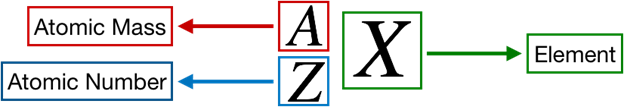# Problem: Fill in the blanks to complete the table.Symbol Z  A  #p  #e-  #n ChargeSi14________1414____S2-____32____________2-Cu2+________________342+____15________1516____Complete the sixth column of the table.

###### FREE Expert Solution

We’re being asked to complete the sixth column of the given table.

Recall that the symbol for an element or ion is as follows:Recall that the number of protons is equal to the atomic number.

For the first, third and fourth rows:

The number of neutrons, #n are already given.

This means that we only need to determine the number of neutrons for the second row

Recall that the mass number is equal to the sum of the number of protons and neutrons.

89% (193 ratings)###### Problem Details
Fill in the blanks to complete the table.

 Symbol Z A #p #e- #n Charge Si 14 ____ ____ 14 14 ____ S2- ____ 32 ____ ____ ____ 2- Cu2+ ____ ____ ____ ____ 34 2+ ____ 15 ____ ____ 15 16 ____

Complete the sixth column of the table.

Frequently Asked Questions

What scientific concept do you need to know in order to solve this problem?

Our tutors have indicated that to solve this problem you will need to apply the Ions concept. You can view video lessons to learn Ions. Or if you need more Ions practice, you can also practice Ions practice problems.

What professor is this problem relevant for?

Based on our data, we think this problem is relevant for Professor Michael's class at UNC.